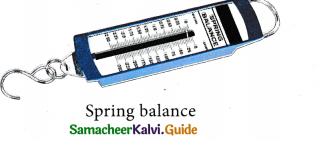Tamilnadu State Board New Syllabus Samacheer Kalvi 9th Science Guide Pdf Chapter 1 Measurement Text Book Back Questions and Answers, Notes.

## Tamilnadu Samacheer Kalvi 9th Science Solutions Chapter 1 Measurement

### 9th Science Guide Measurement Text Book Back Questions and AnswersI. Choose the correct answer :

Question 1.
Choose the correct one.
(a) mm < cm < m < km
(b) mm > cm > m > km
(c) km < m < cm < mm
d) mm > m > cm > km
(a) mm < cm < m < km

Question 2.
Rulers, measuring tapes and metre scales are used to measure
(a) mass
(b) weight
(c) time
(d) length
(d) lengthQuestion 3.
1 metric ton is equal to
(a) 100 quintals
(b) 10 quintals
(c) 1/10 quintals
(d) 1/100 quintals
(b) 10 quintals

Question 4.
Which among the following is not a device to measure mass?
(a) Spring balance
(b) Beam balance
(c) Physical balance
(d) Digital balance
(a) Spring balanceII. Fill in the blanks :

1. Metre is the unit of …………..
length

2. 1 kg of rice is weighed by ……………
beam balance

3. Thickness of a cricket ball is measured by ……………..
vernier caliper4. Radius of a thin wire is measured by …………………
screw gauge

5. A physical balance measures small differences in mass up to ……………….
1mg or less

III. State whether true or false. If false, correct the statement :

1. The SI unit of electric current is kilogram.
False.
Correct statement: The SI unit of electric current is ampere.2. Kilometre is one of the SI units of measurement.
True.

3. In everyday life, we use the term weight instead of mass.
True.

4. A physical balance is more sensitive than a beam balance.
True.5. One Celsius degree is an interval of IK and zero degree Celsius is 273.15 K.
True.

6 . With the help of vernier caliper we can have an accuracy of 0.1 mm and with screw gauge we can have an accuracy of 0.01 mm.
True.

IV. Match the following:

Question 1.

 Column I Column II Length kelvin Mass metre Time kilogram Temperature second

 Column I Column II Length metre Mass kilogram Time second Temperature kelvin

Question 2.

 Column I Column II Screw gauge Vegetables Vernier caliper Coins Beam balance Gold ornaments Digital balance Cricket ball

 Column I Column II Screw gauge Coins Vernier caliper Cricket ball Beam balance Vegetables Digital balance Gold ornamentsV. Assertion and reason type :

Mark the correct answer as :
(a) Both A and R are true but R is not the correct reason.
(b) Both A and R are true and R is the correct reason.
(c) A is true but R is false.
(d) A is false but R is true

Question 1.
Assertion (A) : The scientifically correct expression is “The mass of the bag is 10 kg”
Reason (R) : In everyday life, we use the term weight instead of mass.
(a) Both A and R are true but R is not the correct reason

Question 2.
Assertion (A) : 0° C = 273.16 K. For our convenience we take it as 273K after rounding off the decimal.
Reason (R) : To convert a temperature on the Celsius scale we have to add 273 to the given temperature.
(b) Both A and R are true and R is the correct reasonQuestion 3.
Assertion (A) : Distance between two celestial bodies is measured in terms of light year.
Reason (R) : The distance travelled by the light in one year is one light year.
(d) A is false but R is true
Distance between two celestial bodies is measured in terms of unit.

Question 1.
Define measurement.
Measurement is the process of comparison of the given physical quantity with the known standard quantity of the same nature.

Question 2.
Define a standard unit.
Unit is the quantity of a constant magnitude which is used to measure the magnitudes of other quantities of the same nature.Question 3.
What is the full form of SI system?
The full form of S.I. system is the International System of Units.

Question 4.
Define least count of any device.
Least count is the least measurement possible in a given device.
It is the distance moved by the tip of the screw for a rotation of one division on the head scale.
Least count = [Pitch / No. of head scale divisions]Question 5.
What do you know about pitch of screw gauge?
Pitch of the screw gauge is the distance between two successive screw threads. It is measured by the ratio of distance travelled on the pitch scale to the number of rotations of the head scale.

Question 6.
Can you find the diameter of a thin wire of length 2 m using the ruler from your instrument box?
No, I can not find the diameter of a thin wire of length 2 m using the ruler.Question 1.
Write the rules that are followed in writing the symbols of units in SI system.

•  The units named after scientists are not written with a capital initial letter.
E.g. newton, henry, ampere, and watt.
• The symbols of the units named after scientists should be written by the initial capital letter.
E.g. N for newton, H for Henry, A for ampere, and W for watt.
• Small letters are used as symbols for units not derived from a proper noun.
E.g. m for metre, kg for kilogram.
•  No lull stop or other punctuation marks should be used within or at the end of symbols.
E.g. 50 m and not as 50 m.
•  The symbols of the units are not expressed in plural form.
E.g. 10 kg not as kgs.

Question 2.
Write the need of a standard unit.
A Standard Unit is needed to maintain uniformity in measurements like length, weight, size and distance. Eg: Standard Unit of length is metre.Question 3.
Differentiate mass and weight.
Mass:

1.  Fundamental quantity
2.  Has magnitude alone – scalar quantity
3.  It is the amount of matter contained in a body
4.  Remains the same
5.  It is measured using physical balance
6.  Its unit is kilogram

Weight:

1.  Derived quantity
2.  Has magnitude and direction – vector quantity
3.  It is the normal force exerted by the surface on the object against gravitational pull
4.  Varies from place to place
5.  It is measured using spring balance
6.  Its unit is newton

Question 4.
How will you measure the least count of vernier caliper?
The least count of Vernier Caliper is the ratio of the value of one smallest main scale division to total
a number of Vernier scale divisions.
i.e., L.C. = 0.1mm = 0.01cm
(or) L.C. = 1MD – 1VSD = 1.0 mm – 0.9 mm = 0. 1mm = 0.01 cmQuestion 1.
Explain a method to find the thickness of a hollow teacup.
Step 1 : The Pitch, Least count and the type of zero error of the screw gauge are determined.
Step 2 : The given cup is placed in between two studs.
Step 3 : The head screw using the ratchet arrangement is freely rotated until the given cup is held firmly, but not tightly.
Step 4 : Pitch scale reading (PSR) by the head scale and head scale coincidence (HSC) with the axis of the pitch scale, are found.
Step 5 : The readings are recorded and the experiment for different positions of the given cup is repeated.
Step 6 : The thickness of the cup is calculated using the formula P.S.R + (HSC × L.C)
Step 7 : Then the average of the last column of the table, is found. Hence the thickness of a hollow tea cup = ………….. mm.

Question 2.
How will you find the thickness of a one rupee coin?
Step 1 : The Pitch, Least count and the type of zero error of the screw gauge are determined.
Step 2 : The given coin is placed in between two studs.
Step 3 : The head screw using the ratchat arrangement is freely rotated until given one rupee coin is held firmly, but not tightly.
Step 4 : Pitch scale reading (PSR) by the head scale and head scale coincidence (HSC) with are axis of the pitch scale are found.
Step 5 : The reading are recorded and the experiment for different positions of the given coin is repeated.
Step 6 : The thickness of the coin is computed using the formula P.S.R + (HSC x L.C )
Step 7 : Then the average of the last column of the table is found.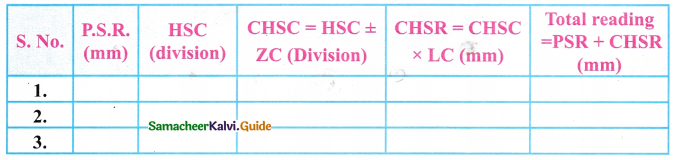mean = …………….. mm
Hence the thickness of a one rupee coin = ……………….. mmIX. Numerical Problems :

Question 1.
Inian and Ezhilan argue about the light year. Inian tells that it is 9.46 x 1015m and Ezhilan argues that it is 9.46 x 1012 km. Who is right? Justify your answer.
(Inian is correct)
Light travels 3 × 108m in one second or 3 Lakhs kilometre in one second.
In one year we have 365 days.
The total number of second in one year is equal to 365 x 24 x 60 x 60
Distance travelled by light in 1 year = (3.153 x 107) x (3 x 108)
= 9.46 x 1015m

Question 2.
The main scale reading while measuring the thickness of a rubber ball using Vernier caliper is 7 cm and the Vernier scale coincidence is 6. Find the radius of the ball.
MSR = 7 cm
VC = 6cm
LC = 0.1mm = 0.1cm
Diameter = DR = MSR + (VC X LC)
= 7 + 0.06 cm
Diameter D = 7.06 cm
Radius R = $$\frac{D}{2}=\frac{7.06}{2}$$ = 0.035 m
The radius of the ball = 0.0353 m.Question 3.
Find the thickness of a five rupee coin with the screw gauge, if the pitch scale reading is 1 mm and its head scale coincidence is 68.
PSR = 1 mm = 1 x 10-3m
HSC = 68
LC = 0.01 mm =0.01 × 10-3m
Total reading = PSR + (HSC × LC)
∴Thickness of the five rupee coin = 1 × 10-3 + (68 × 0.01 × 10-3 )m
∴ Thickness of the five rupee coin = 1.68 × 10-3m = 1.68mm

Question 4.
Find the mass of an object weighing 98 N.
W = mg
W =98 N
g = 9.8 m/s2
m = $$\frac{\mathrm{W}}{\mathrm{g}}=\frac{98}{9.8}$$ = 10 kgIntext Activities

ACTIVITY – 1

Using Vernier caliper find the outer diameter of your pen cap.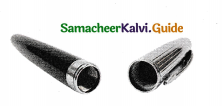Aim : To find the outer diameter of the pen cap.
Materials required : Vernier caliper, pen cap.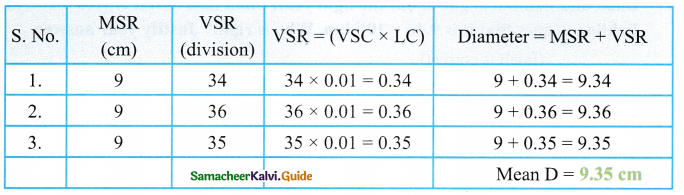Result: The outer diameter of the pen cap = 9.35 cm
[End of the activity]ACTIVITY – 2

Determine the thickness of a single sheet of your science textbook with the help of a Screw gauge.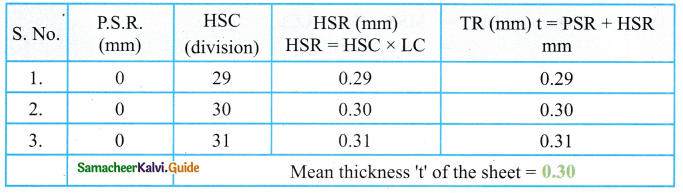LC = Least Count
Result : The thickness of the single sheet = 0.30
End of the activity

I. Choose the correct answer :

Question 1.
Length is ……………….
(a) The amount of matter in an object
(b) The amount of space an object takes up.
(c) The distance between two points.
(d) The amount of stuff in an object
(c) The distance between two points

Question 2.
Mass is ………………….
(a) The distance between two points
(b) The distance between three points
(c) The amount of matter contained in an object
(d) The amount of space an object occupies.
(c)The amount of matter contained in an objectQuestion 3.
Unit used to measure length
(a) metre
(b) litre
(c) gram
(d) cubic metre (m3)
(a) metre

Question 4.
Unit which is used to measure mass
(a) ml
(b) 1
(c) cm
(d) gram
(d) gramQuestion 5.
How many metres are there in 1 nanometer?
(a) 10-10m
(b) 10-9m
(c) 109m
(d) 1010m
(b) 10-9m

Question 6.
What unit will you use to measure the length of our classroom?
(a) km
(b) m
(c) cm
(d) mm
(b) mQuestion 7.
The Kelvin is the basic unit of ………………..
(a) temperature
(b) mass
(c) length
(d) volume
(a) temperature

Question 8.
……………….consists of ‘U’ shape metal frame
(a) Screw gauge
(b) Vernier caliper
(c) Beam balance
(d) Spring balance
(a) screw gaugeQuestion 9.
Least count of a vernier caliper is ……………. cm.
(a) 1
(b) 0.1
C) 0.01
(d) 0.001
(c) 0.01

Question 10.
If no object is placed on the hook, then the pointer of the spring balance reads ………………….
(a) 3
(b) 2
(c) 1
(d) 0
(d) 0Question 11.
SI unit of mass and weights are ………………….
(a) kg, N
(b) N, kg
(c) K, N
(d) N, K
(a) kg, N

Question 12.
Units named after scientists …………………..
(a) lowercase
(b) upper case
(c) both (a) and (b)
(d) neither (a) or (b)
(a) lower caseQuestion 13.
An instrument that is used to measure the diameter of a cricket ball is ………………….
(a) Screw gauge
(b) Meter scale
(c) Vernier caliper .
(d) Spring balance
(a) Vernier caliper

Question 14.
Distance between Chennai and Kanyakumari can be found in
(a) Kilometres
(b) Metres
(c) Centimetres
(d) Millimetres.
(a) KilometresII. Fill in the blanks :

1. The precision of vernier calipers is ……………. mm.
(a) 0.1mm

2. The gravity accelerates an object, the distance fallen is proportional to …………………
time squared

3. SI unit of electric current is ………………
ampere4. Larger unit for measuring time is ………………..
millennium

5. The value of an astronomical unit is ……………………
1.496 x 1011

6. Mass is a …………….. quantity.
scalarIII. State whether true or false. If false, correct the statement:

1. The precision of screw gauge is 0.001 cm.
True.

2. The unit of amount of substance is candela
False.
Correct statement: The unit of amount of substance is mole.

3. The symbol for the units derived from the names of scientists are written in capital letter
True.4. Yard was used as the unit of length.
True

5. Micron is also known as micro-metre
True

6. A vemier caliper using the scale invented by Galileo.
False.
Correct statement: A vernier caliper using the scale invented by Pierre Vernier.7. The SI unit of mass is kg.
True.

8. Weight has both magnitude and direction.
True.IV. Match the following:

Question 1.

 Column I Column II FPS Metre, kilogram and second CGS Foot, pound and second MKS or SI centimetre, gram and second

 Column I Column II FPS Foot, pound and second CGS centimetre, gram and second MKS or SI Metre, kilogram and second

Question 2.

 Column-I Column-II 10 years 1 year 10 centuries 1century(100 years) 10 decades 1 millennium 365.24 days 1 decade

 Column-I Column-II 10 years 1 decade 10 centuries 1 millennium 10 decade 1century(100 years) 365.24 days 1 yearQuestion 3.

 Column-I Column-II Electric Current radian Luminous intensity ampere Angle steradian Solid angle candela

 Column-I Column-II Electric Current ampere Luminous intensity candela Angle radian Solid angle steradian

Question 4.

 Column-I Column-II Length s Mass m Time k Temperature kg

 Column-I Column-II Length m Mass kg Time s Temperature kQuestion 5.

 Column-I Column-II Millimeter 10-15 m Nanometer 10-3m Angstrom 10-9m Fermi 10-10m

 Column-I Column-II Millimeter 10-3m Nanometer 10-9m Angstrom 10-10m Fermi 10-15 m

Question 6.

 Column-I Column-II Temperature Bearm balance Mass Ruler Length Digital clock Time Thermometer

 Column-I Column-II Temperature Thermometer Mass Bearm balance Length Ruler Time Digital clockV. Assertion and reason type :

Question 1.
Assertion (A) : Light year and wave length both measure distance
Reason (R) : Both have dimensions of time.
(a) Both A and R are true but R is not the correct explanation of A.
(b) Both A and R are true and R is the correct explanation of A.
(c) A is true but R is false.
(d) A is false but R is true.
(c) A is true but R is false

Question 2.
Assertion (A) : Density is a derived physical quantity
Reason (R) : Density cannot be derived from the fundamental physical quantities.
(a) Both A and R are true but R is not the correct explanation of A.
(b) Both A and R are true and,R-is the correct explanation of A.
(c) A is true but R is false. .
(d) A is false but R is true.
(c) A is true but R is false
Correct statement: Density can be derived from mass and volume.Question 3.
Assertion (A) : Mass, Length and Time are fundamental physical quantities.
Reason (R) : They are independent of each other.
(a) Both A and R are true but R is not the correct explanation of A.
(b) Both A and R are true and R is the correct explanation of A.
(c) A is true but R is false.
(d) A is false but R is true.
b) Both A and R are true and R is the correct explanation of A

Question 4.
Assertion (A) : The SI system of units is the improved system of units for measurement.
Reason (R) : The SI unit of mass is kilogram.
(a) Both A and R are true but R is not the correct reason.
(b) Both A and R are true and R is the correct reason.
(c) A is true but R is false.
(d) A is false but R is true.
(b) Both A and R are true and R is the correct reasonQuestion 5.
Assertion (A) : The skill of estimation is important for all of us in our daily life.
Reason (R) : The skill of estimation reduces our consumption of time.
a) Both A and R are true but R is not the correct reason.
b) Both A and R are true and R is the correct reason.
c) A is true but R is false. ,
d) A is false but R is true.
(b) Both A and R are true and R is the correct reason

VI. Comprehensive type :

(a) The speed of a body gives us an idea of how slow or fast that a body is moving. Speed of a body is the distance travelled by it per unit time. The SI unit of speed is metre per second. It is a scalar quantity. The speed of a running cab at any instant of time is shown by an instrument called, ’speedometer’ and the distance travelled by a car is measured by another instrument called, ‘odometer’.

Question 1.
Which the following is not a correct unit of speed?
(a) cm/s
(b) m/s
(e) km/h
(d) km/s.
(d) km/s

Question 2.
If the distance travelled by the cab in 3 hours is 120 km, then its speed will be
(a) 40 m/s
(b) 40 km/s
(c) 40 km/h
(d) 40 km/min
(c) 40 km/hQuestion 3.
The formula for finding the speed of the cab is
(a) Distance = speed x time
(b) velocity = distance x time
(c) time = distance x velocity
(d) None of these.
(a) Distance = speed x time

Mass is the amount of matter contained in an object. Measurement of mass helps us to distinguish between lighter and a heavier body. Beam-Balance, spring balance and electronic balance are used to measure mass of different objects. The SI unit of mass is kilogram (kg). But different units are Used to measure the mass of different objects depending upon their weight, e.g. weight (mass) of a tablet is measured in milligrams (mg), weight of a student is measured in kilogram (kg), and weight of a truck with goods is measured in metric tons. 1 metric ton is equal to 10 quintals and 1 quintal is equal to 100 kg. 1 gram is equal to 1000 mg.

Question 1.
The value of 1 metric ton is equal to
(a) 1000 kg
(b) 10 quintals
(c) 1000,000 g
(d) 100 kg
(a) 1000 kg (or) (b) 10 quintalsQuestion 2.
How will you measure weight of a tablet?
(a) kg
(b) g
(c) mg
(d) none of these.
(c) mg

Question 1.
Write the units which are used to measure long distances.
km, AU, light-year, parsec.

Question 2.
Define Astronomical unit.
AU is defined as the average distance between the earth and the sun.
1 AU = 1.496 × 1011m.

Question 3.
Define the light year.
The distance travelled by light in one year in vacuum. 1 light-year = 9.46 × 1015m.Question 4.
Convert the temperature from Fahrenheit into Celsius & Kelvin.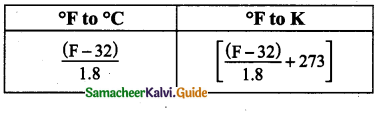Question 5.
Convert 100°C into Kelvin.
100 + 273 = 373 K. ie. °C + 273

Question 6.
Convert 112°F into K.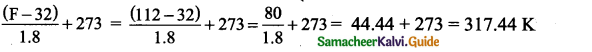Question 7.
Write the principle of screw gauge.

• When a screw is rotated in a nut, the distance moved by the tip of the screw is directly proportional to the number of rotations given.
• Hence the principle of the screw is considered as the principle of screw gauge.

Question 8.
What are the kinds of units?

1. Fundamental or basic units
2. Derived unitsQuestion 9.
Give some examples of fundamental units.
The examples of fundamental units are kg for mass,m for length, s for time.

Question 10.
Give some examples of derived units.
The units of area, volume, density.

Question 11.
What is the standard unit of weight?
Newton is the standard unit of weight.Question 12.
What is the standard unit of mass?
kilogram is the standard unit of mass.

Question 13.
Define Mass.
Mass is the amount of matter contained in a body.

Question 14.
Define Weight.
The force with which the earth attracts a body towards its center is called weight.Question 15.
What is the SI unit of temperature?
Kelvin is the SI unit of temperature.

Question 16.
What is the measuring unit of the thickness of a plastic carry bag?
1 micron = 10-6m (or) μm.Question 1.
Write temperature conversion.
Temperature Conversion (Exact)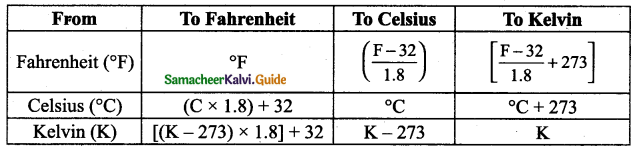Question 2.
Write about the positive zero error in screw gauge instrument.
When the plane surface of the screw and the opposite plane stud on the frame are brought into contact, if the zero of the head scale lies below the pitch scale axis, the zero error is positive. For example, the 5th division of the head scale coincides with the pitch scale axis, then the zero error is positive and is given by Z.E = + (n × LC) where ‘n’ is the head scale coincidence. In this case, Zero error = + (5 × 0.01) = 0.05 mm. So the zero correction is – 0.05 mm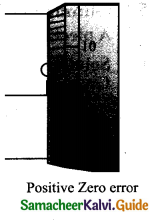Question 3.
Write SI units for the fundamental quantity.

 Basic Quantity Unit Length metre Mass kilogram Time second Electric current ampere Temperature kelvìn Amount of substance mole Luminous intensity candela

Question 4.
Convert the following units in metre.

 Smaller units Value in metre Centimeter (cm) 10-2 m Millimeter (mm) 10-3 m micron or µm 10-6 m nanometre (nm) 10-9 m angstrom (A) 10-10 m fermi (f) 10-15 mQuestion 5.
Draw and mark the parts of vernier caliper
PARTS Marked in the Vernier caliper

1. Lower fixed jaw
2. Upper fixed jaw
3. Lower movable jaw
4. Vernier scale
5. Retainer
6. Main scale
7. Depth probe.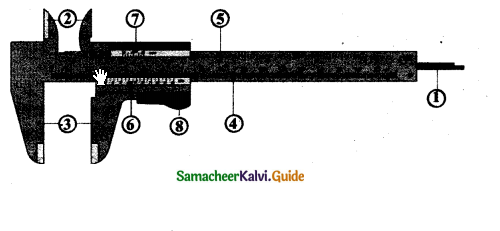IX. Numerical Problems :

Question 1.
A piece of iron of volume 40cm3 whose density is 6.8g/cm3. Find the mass of iron.
Given, density of iron, D = 6.8g/cm3
volume of iron, V = 40 cm3
mass of iron, M = V × D [∴ mass = volume × density]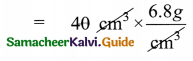m = 272.0g.

Question 2.
Solve : The mass of 40 apples in a box is 5 kg.
(i) Find the mass of a dozen of them.
(ii) Express the mass of one apple in gram.
(i)  40 apple = 5 kg 5000 g
1 apple =$$\frac{500 \not 0}{4 \not \emptyset}$$
1 apple 125 g
∴ 1 dozen 12 apples
12 apples 125 × 12 g
12 apples 1500 g.

(ii)  40 apples = 5000 g

1 apple = $$\frac{5000}{40} \mathrm{~g}$$

1 apple = 125 g
The mass of 1 apple = 125 gQuestion 1.
How will you find Zero Error of the screw gauge?
Zero Error of a screw gauge :
When the plane surface of the screw and the opposite plane stud on the frame area brought into contact, if the zero of the head scale coincides with the pitch scale axis there is no zero error.

Positive zero error:
When the plane surface of the screw and the opposite plane stud on the frame are brought into contact, if the zero of the head scale lies below the pitch scale axis, the zero error is positive. For example, the 5th division of the head scale coincides with the pitch scale axis, then the zero error is positive and is given by
Z.E = + (n x LC) where ‘n’ is the head scale coincidence. In this case, Zero error = + (5 x 0.01) = 0.05mm. So the zero correction is -0.05 mm.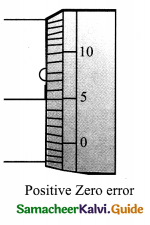Negative zero error:
When the plane surface of the screw and the opposite plane stud on the frame are brought into contact, if the zero of the head scale lies above the pitch scale axis, the zero error is negative. For example, the 95th division coincides with the pitch scale axis, then the zero error is negative and is given by
ZE = -(100-n) × LC
ZE = – (100 – 95) × LC
= -(5 × 0.01)
= – 0.05 mm
The zero correction is + 0.05mm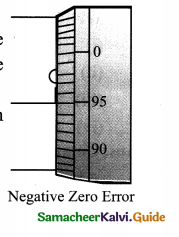Question 2.
How will you find Zero Error of Vernier Caliper? Explain.
Zero error:

• Unscrew the slider and move it to the left, such that both the jaws touch each other. Check whether the zero marking of the main scale coincides with that of the Vernier scale.
• If they are not coinciding with each other, the instrument is said to posses zero error. Zero error may be positive or negative.
• If the zero mark of the Vernier is shifted to the right, it is called positive error.
• On the other hand, if the Vernier zero is shifted to the left of the main scale zero marking, then the error is negative.

Positive zero error:

• From the figure you can see that zero of the vernier scale is shifted to the right of zero of the main scale.
• In this case the reading will be more than the actual reading.
• Hence, this error should be corrected. In order to correct this error, find out which vernier division is coinciding with any of the main scale divisions.
• Here, fifth vernier division is coinciding with a main scale division.
• So, positive zero error = +5 × LC = +5 × 0.01 = 0.05 cm.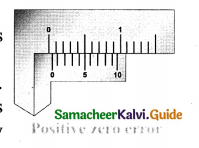Negative zero error:

• You can see that zero of the vernier scale is shifted to the left of the zero of the main scale.
• So, the obtained reading will be less than the actual reading.
• To correct this error we should first find which vernier division is coinciding with any of the main scale divisions, as we found in the previous case.
• In this case, you can see that sixth line is coinciding. But, to find the negative error, we can count backward (from 10).
• So, the 4th line is coinciding. Therefore, negative zero error = -4 x LC = -4 x 0.01 = -0.04 cm.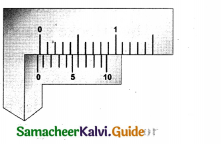Question 3.
Write short note on the following :
(i) Common balance
(ii) Physical balance Digital balance Spring balance
Common (beam) balance :
A beam balance compares the sample mass with a standard reference mass (Standard reference masses are 5g, 10g, 20g, 50g, 100g, 200g, 500g, 1kg, 2kg, 5kg). This balance can measure mass accurately up to 5 g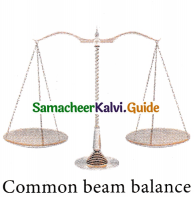Physical balance:
This balance is used in labs and is similar to the beam balance but it is a lot more sensitive and can measure mass of an object correct to a milligram.
The standard, reference masses used in this physical balance are 10 mg, 20 mg, 50 mg, 100 mg, 200 mg, 500 mg, 1 g, 2g, 5 g, 10 g, 20 g, 50 g, 100g, and 200 g.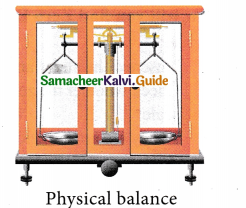Digital balance:
Nowadays, for accurate measurements digital balances are used, which measure mass accurately even up to a few milligrams, the least value being 10 mg (Figure 1.11). This electrical device is easy to handle and commonly used in jewellery shops and labs.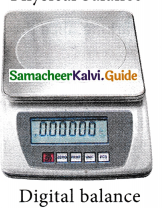Spring balance:
This balance helps us to find the weight of an object. It consists of a spring fixed at one end and a hook attached to a rod at the other end. It works by ‘Hooke’s law’ which states that the addition of weight produces a proportional increase in the length of the spring. A pointer is attached to the rod which slides over a graduated scale on the right. The spring extends according to the weight attached to the hook and the pointer reads the weight of the object on the scale.